# Acceleration

## Terminology

Acceleration is the change in velocity over time. The change in velocity is caused by a force and there is:

[2.2a]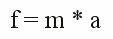or      [2.2b]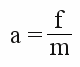or      [2.2c]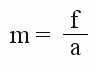[2.3a]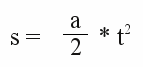or      [2.3b]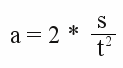or      [2.3c][2.4a]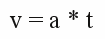or      [2.4b]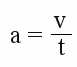or      [2.4c]Where is:
s - distance, t - time, v - velocity, f - force, m - mass, a - acceleration
The above given correlations assume that the speeds are clearly smaller than the speed of light and the mass of the object is constant. The maximum speed of the fastest car on earth is 1227.98 km/h. Because light is traveling with 1,079,252,848.8 km/h through the vacuum, condition number one is fulfilled. While driving, a mass change of cars is mostly caused by combustion of fuel. Assuming a compact car with a mass of 700kg and a tank with 20L capacity, the weight change is 3%. If you have a look at the acceleration from 0 to 100 km/h, which is done within less than one minute, just a fraction of those 20L is combusted and the loss of weight is insignificant. Condition number two is also fulfilled.
Figure 1:

## Distance vs. time plot

If the force affecting an object is constant, the result of the plot is a parabola. The parabola's slope represents the velocity of the object.

## In practice

Car manufacturers declare the acceleration of their vehicles indirectly: They publish the time needed to accelerate from 0 to 100 km/h respectively from 0 to 60mph. By using formula (4b) you can calculate the average acceleration based on the given values.
Besides the acceleration induced by throttle pedal, there are some more situations where acceleration operates. Braking means accelerating, too! The maximum acceleration values of a full brake application are consistently higher than during the speed-up. Even a compact car can stop within 50m from a speed of 100 km/h. Just a few roadsters can accelerate up to 100km/h within this distance. Even while the speedometer shows the same value, acceleration can occur. While driving through a turn, the travel direction and thus the velocity vector is altered. The passengers will realize this kind of acceleration by the counterforce, the centrifugal force. Besides this, every rough road accelerates a car in vertical direction.

The following table lists some objects with their characteristic acceleration values:

 Object a in m/s2 Remark Fiat 126 with original combustion engine (17kW) 0.59 while accelerating from 0km/h to 100km/h within 47 seconds Porsche Carrera GT (450kW) 7.31 while accelerating from 0km/h to 100km/h within 3.8 seconds Fiat 126 with original combustion engine (17kW) 7.41 while slowing down from 100km/h to 0km/h within 52 meters. Higher than the Porsche during speed-up... Thrust SSC 17.36 Average acceleration from 0km/h to 1000km/h within 16 seconds. Space Shuttle 40 Starting from launch pad with burning boosters.

## From time specification 0-100km/h to the average acceleration

The conversion is based on formula (4b):
v=100km/h according 100 / 3.6 m/s = 27.78m/s. Our compact car needs t=47s to speed-up.
a = 27.78 / 47 m/s2 = 0.59 m/s2.

## From stopping distance 100-0km/h to the average acceleration

Inserting formula (4C) in formula (3a) results in:
s=a/2 * (v/a)2 = a/2 * v2/a2 = v2/(2*a).